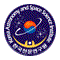# Korean VLBI Network (KVN)

## Antenna Position Measurement

The IVP(invariant point) determination of KVN antennas was done by using a GPS system. Two GPS antennas were installed at both sides of elevation gears and measured their positions for a day. Each location of receivers were determined, and then the IVP was calculated using those two GPS antenna positions and their offsets from the IVP (figure 1).Figure 1. IVP determination of KVN antennas using two GPS antennas

The KVN antenna positions are described in the section, "Antenna site".

## Atmospheric Model Evaluation

A dual-band GPS receiver installed at each KVN site is used to evaluate an atmospheric model in order to get a priori (or post calibration) for a correlation and for high precision astrometry. The total wet delay and TEC (total electron content) estimations calculated by GPS measurements help to calibrate the differences in excess path length, passing through different atmosphere.Figure 2. GPS applications for atmospheric calibrationKorean VLBI Network